# 树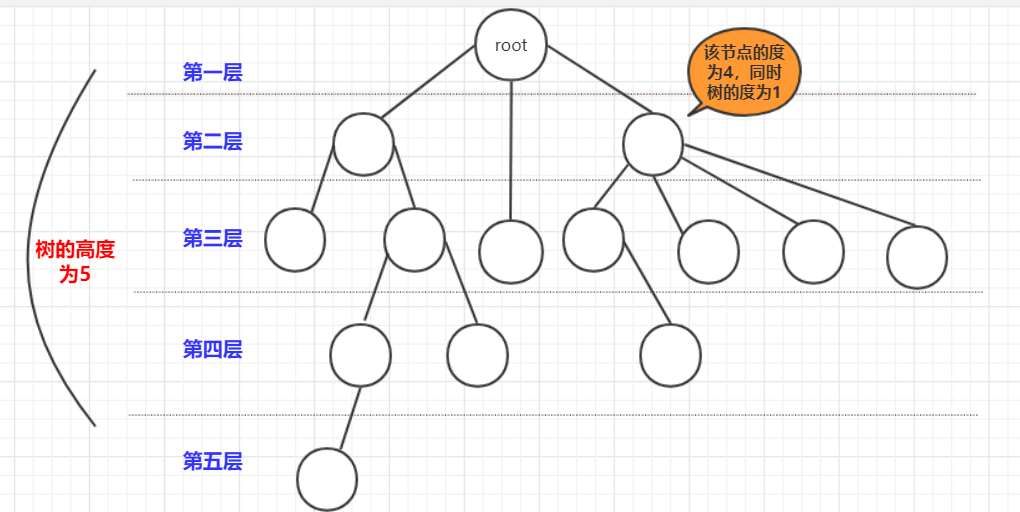• 树是递归的，将树的任何一个节点以及节点下的节点都能组合成一个新的树。并且很多操作基于递归完成。
• 根节点： 最上面的那个节点(root)，根节点没有前驱节点，只有子节点（0个或多个都可以）
• 层数： 一般认为根节点是第1层(有的也说第0层)。而树的高度就是层数最高(上图层数开始为1)节点的层数
• 节点关系: 父节点：就是链接该节点的上一层节点,孩子节点:和父节点对应，上下关系。而祖先节点是父节点的父节点(或者祖先）节点。兄弟节点：拥有同一个父节点的节点们！
• 度： 节点的度就是节点拥有孩子节点的个数(是孩子不是子孙).而树的度(最大)节点的度。同时，如果度大于0就成为分支节点,度等于0就成为叶子节点（没有子孙）。

• 树的节点数=所有节点度数+1.
• 度为m的树第i层最多有mi-1个节点。(i>=1)
• 高度而h的m叉树最多（mh-1）/(m-1)个节点(等比数列求和)
• n个节点的m叉树最小高度[logm(n(m-1)+1)]

# 二叉树

• 一：度为2的的树必须有三个节点以上，二叉树可以为空。
• 二：二叉树的度不一定为2：比如说斜树。
• 三：二叉树有左右节点区分，而度为2的树没有左右节点的区分。

• 满二叉树。高度为n的满二叉树有2n-1个节点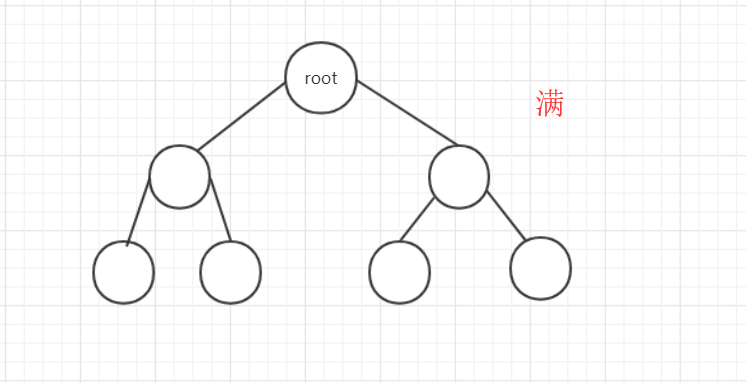• 完全二叉树：上面一层全部满，最下一层从左到右顺序排列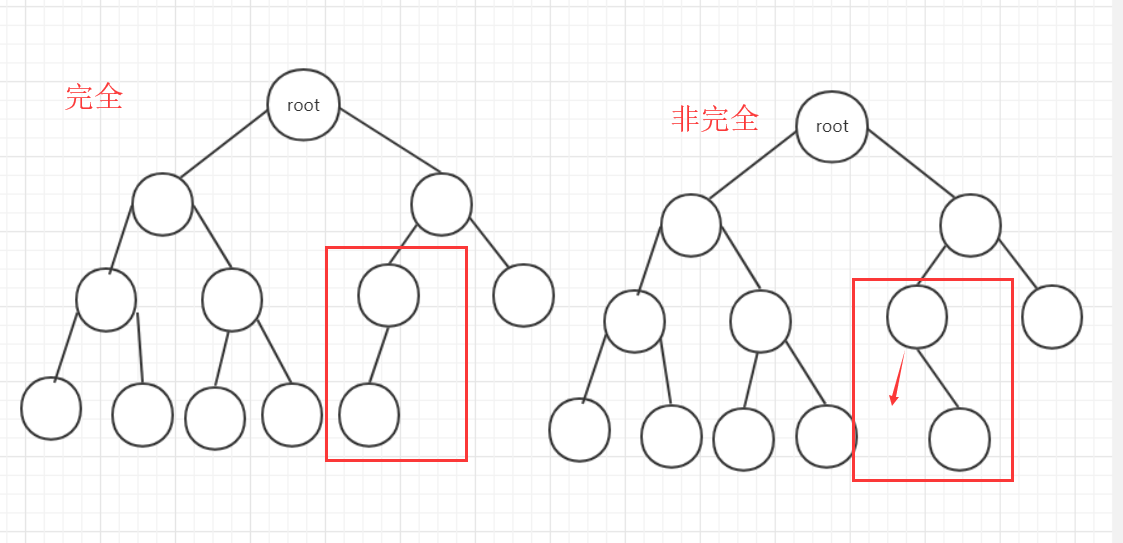• 二叉排序树：树按照一定规则插入排序(本文详解)。
• 平衡二叉树：树上任意节点左子树和右子树深度差距不超过1.

• 非空二叉树叶子节点数=度为2的节点树+1.本来一个节点如果度为1.那么一直延续就一个叶子，但如果出现一个度为2除了延续原来的一个节点，会多出一个节点需要维系。所以到最后会多出一个叶子
• 非空第i层最多有2i-1个节点。
• 高为h的树最多有2h-1个节点(等比求和)。
• 完全二叉树若从左往右，从上到下编号如图：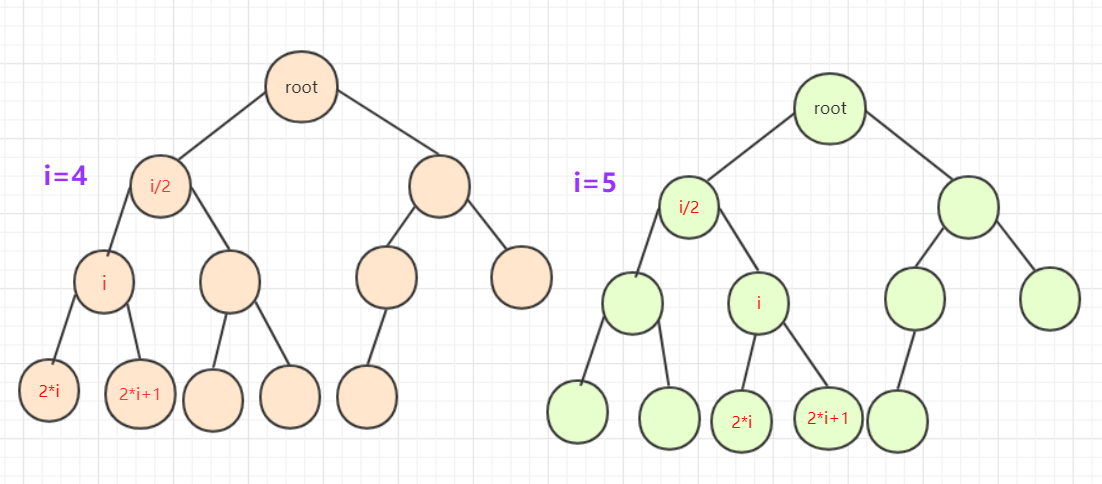# 二叉排序(搜索)树

## 概念

• 从任意节点开始，节点左侧节点值总比节点右侧值要小。
例如。一个二叉排序树依次插入15，6，23，7，4，71，5，50会形成下图顺序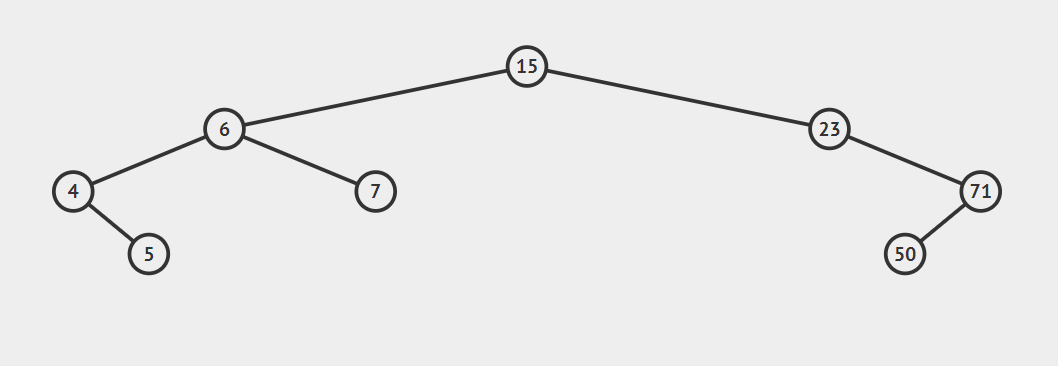## 构造

• 对于node需要这些属性：left，right，和value。其中left和right是左右指针，而value是储存的数据，这里用int 类型。

node类构造为：

class node {//结点
public int value;
public node left;
public node right;
public node()
{
}
public node(int value)
{
this.value=value;
this.left=null;
this.right=null;
}
public node(int value,node l,node r)
{
this.value=value;
this.left=l;
this.right=r;
}
}


public class BinarySortTree {
node root;//根
public BinarySortTree()
{root=null;}
public void makeEmpty()//变空
{root=null;}
public boolean isEmpty()//查看是否为空
{return root==null;}
//各种方法
}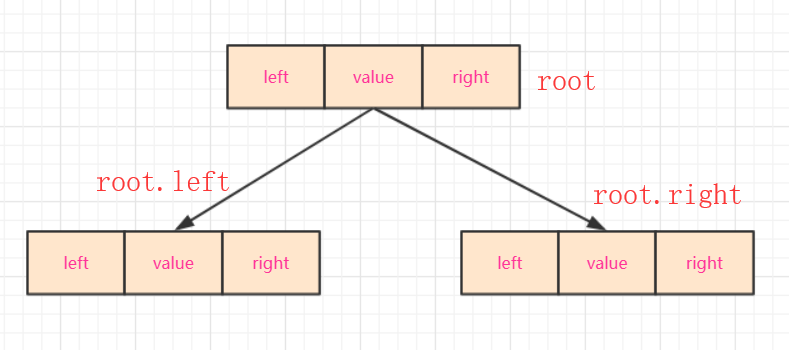## 主要方法

• 既然已经构造号一棵树，那么就需要实现主要的方法。因为二叉排序树中每个节点都能看作一棵树。所以我们创建方法的是时候加上节点参数(也就是函数对每一个节点都能有效)

### findmax(),findmin()

findmin()找到最小节点：

• 因为所有节点的最小都是往左插入，所以只需要找到最左侧的返回即可。

findmax()找到最大节点：

• 因为所有节点大的都是往右面插入，所以只需要找到最右侧的返回即可。
代码使用递归函数
public node findmin(node t)//查找最小返回值是node，调用查看结果时需要.value
{
if(t==null) {return null;}
else if(t.left==null) {return t;}
else return(findmin(t.left));
}
public node findmax(node t)//查找最大
{
if(t==null) {return null;}
else if(t.right==null) {return t;}
else return(findmax(t.right));
}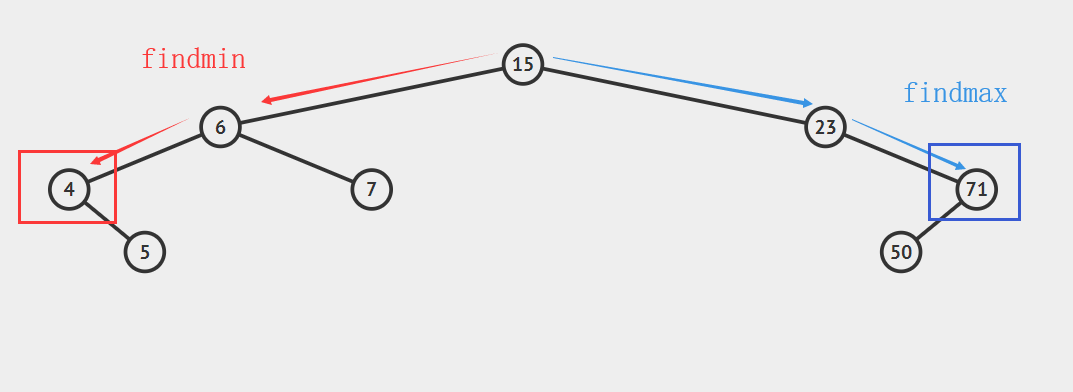### isContains(int x)

• 假设我们我们插入x，那么如果存在x我们一定会在查找插入路径的过程中遇到x。因为你可以如果已经存在的点，再它的前方会走一次和它相同的步骤。也就是说前面固定，我来1w次x，那么x都会到达这个位置。那么我们直接进行查找比较即可！
public boolean isContains(int x)//是否存在
{
node current=root;
if(root==null) {return false;}
while(current.value!=x&&current!=null)
{
if(x<current.value) {current=current.left;}
if(x>current.value) {current=current.right;}
if(current==null) {return false;}//在里面判断如果超直接返回
}
//如果在这个位置判断是否为空会导致current.value不存在报错
if(current.value==x) {return true;}
return false;
}


### insert(int x)

public node insert(int x)// 插入 t是root的引用
{
node current = root;
if (root == null) {
root = new node(x);
return root;
}
while (current != null) {
if (x < current.value) {
if (current.left == null) {
return current.left = new node(x);}
else current = current.left;}
else if (x > current.value) {
if (current.right == null) {
return current.right = new node(x);}
else current = current.right;
}
}
return current;//其中用不到
}

• 比如说上面结构插入51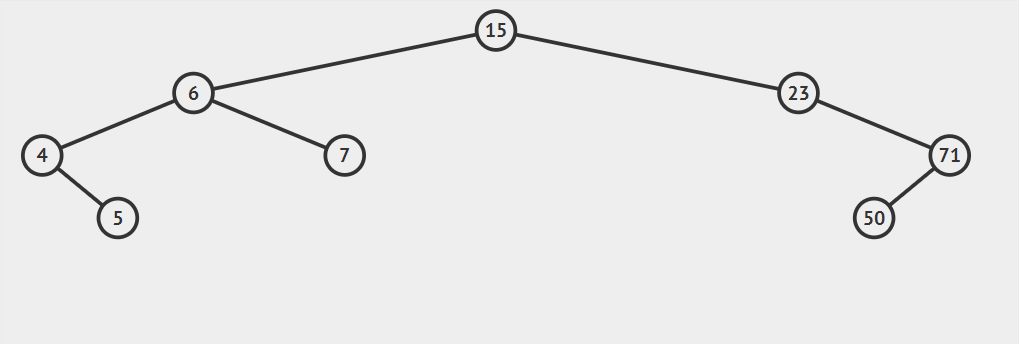### delete(int x)

• 先找到这个点。这个点用这个点的子树可以补上的点填充该点，然后在以这个点为头删除替代的子节点（调用递归）然后在添加到最后情况（只有一个分支，等等）。
• 首先要找到移除的位置，然后移除的那个点分类讨论，如果有两个儿子，就选右边儿子的最左侧那个点替代，然后再子树删除替代的那个点。如果是一个节点，判断是左空还是右空，将这个点指向不空的那个。不空的那个就替代了这个节点。入股左右都是空，那么他自己变空null就删除了。

• 这种情况不需要考虑，直接删除即可。(途中红色点)。另节点=null即可。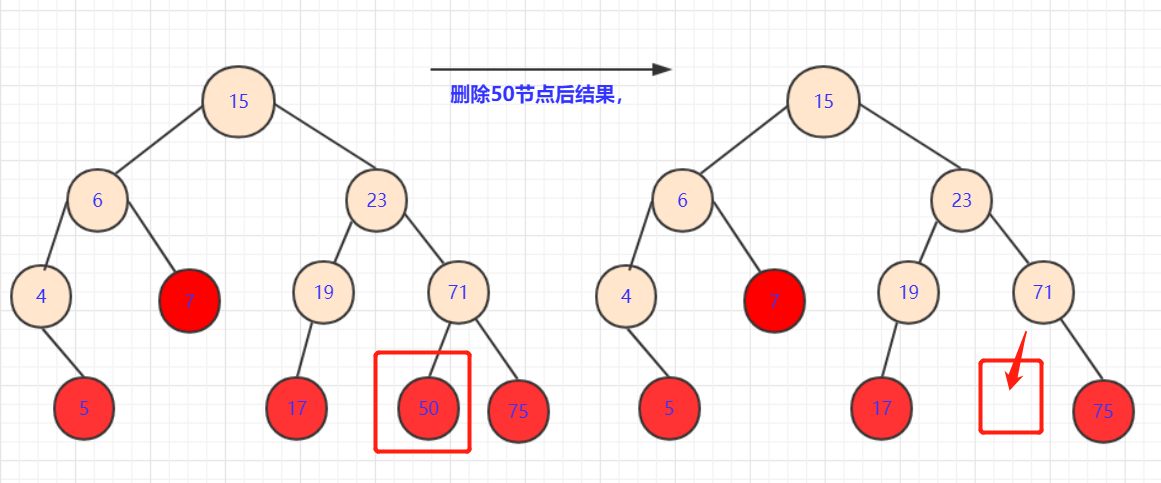• 此种情况也很容易，直接将删除点的子节点放到被删除位置即可。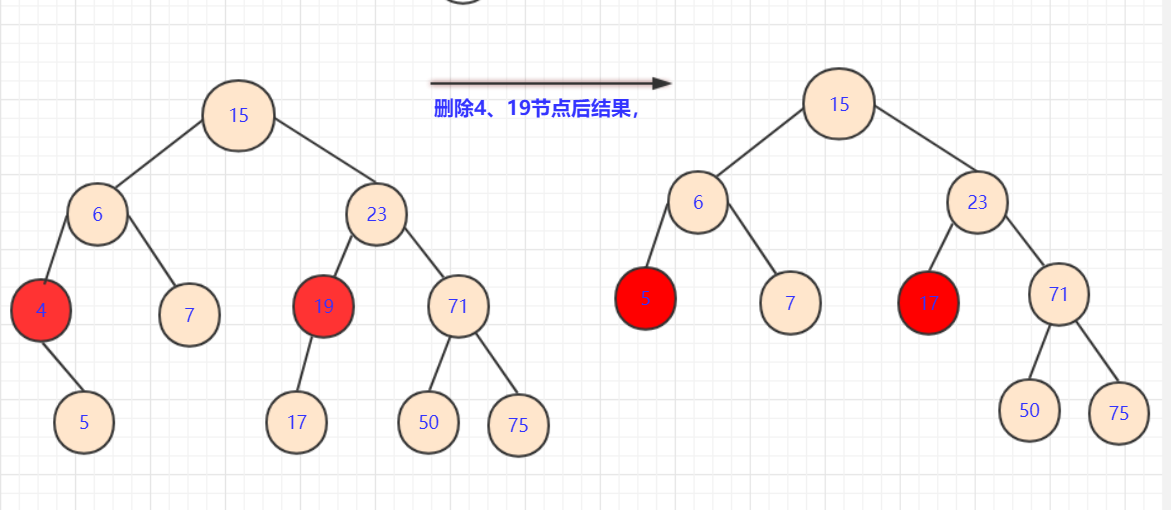• 这种情况相对是复杂的。因为这涉及到一个策略问题• 如果拿19或者71节点填补。虽然可以保证部分侧大于小于该节点，但是会引起合并的混乱.比如你若用71替代23节点。那么你需要考虑三个节点(19,50,75)之间如何处理，还要考虑他们是否满，是否有子女。这是个极其复杂的过程。
• 首先，我们要分析我们要的这个点的属性：能够继承被删除点的所有属性。如果取左侧节点(例如17)那么首先能满足所有右侧节点都比他大（右侧比左侧大）。那么就要再这边选一个最大的点让左半枝都比它小。我们分析左支最大的点一定是子树最右侧
• 如果这个节点是最底层我们很好考虑，可以直接替换值，然后将最底层的点删除即可。但是如果这个节点有左枝。我们该怎么办？
• 这个分析起来也不难，用递归的思想啊。我们删除这个节点，用可以满足的节点替换了。会产生什么样的后果？• 多出个用过的19节点，转化一下，在左子树中删除19的点！那么这个问题又转化为删除节点的问题，查找左子树中有没有能够替代19这个点的。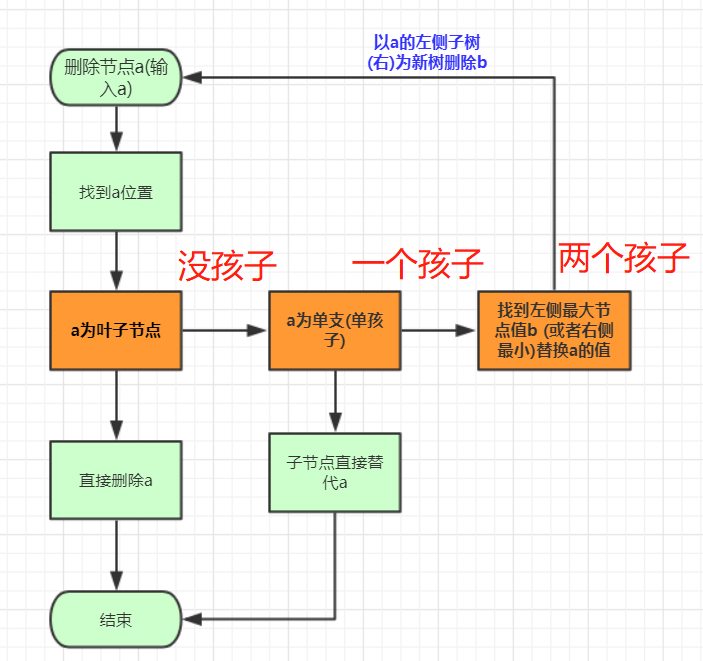public node remove(int x, node t)// 删除节点
{
if (t == null) {
return null;
}
if (x < t.value) {
t.left = remove(x, t.left);
} else if (x > t.value) {
t.right = remove(x, t.right);
} else if (t.left != null && t.right != null)// 左右节点均不空
{
t.value = findmin(t.right).value;// 找到右侧最小值替代
t.right = remove(t.value, t.right);
} else // 左右单空或者左右都空
{
if (t.left == null && t.right == null) {
t = null;
} else if (t.right != null) {
t = t.right;
} else if (t.left != null) {
t = t.left;
}
return t;
}
return t;
}


## 完整代码

package 二叉树;

import java.util.ArrayDeque;
import java.util.Queue;
import java.util.Stack;

public class BinarySortTree {
class node {// 结点
public int value;
public node left;
public node right;

public node() {
}

public node(int value) {
this.value = value;
this.left = null;
this.right = null;
}

public node(int value, node l, node r) {
this.value = value;
this.left = l;
this.right = r;
}
}

node root;// 根

public BinarySortTree() {
root = null;
}

public void makeEmpty()// 变空
{
root = null;
}

public boolean isEmpty()// 查看是否为空
{
return root == null;
}

public node findmin(node t)// 查找最小返回值是node，调用查看结果时需要.value
{
if (t == null) {
return null;
} else if (t.left == null) {
return t;
} else
return (findmin(t.left));
}

public node findmax(node t)// 查找最大
{
if (t == null) {
return null;
} else if (t.right == null) {
return t;
} else
return (findmax(t.right));
}

public boolean isContains(int x)// 是否存在
{
node current = root;
if (root == null) {
return false;
}
while (current.value != x && current != null) {
if (x < current.value) {
current = current.left;
}
if (x > current.value) {
current = current.right;
}
if (current == null) {
return false;
} // 在里面判断如果超直接返回
}
// 如果在这个位置判断是否为空会导致current.value不存在报错
if (current.value == x) {
return true;
}
return false;
}

public node insert(int x)// 插入 t是root的引用
{
node current = root;
if (root == null) {
root = new node(x);
return root;
}
while (current != null) {
if (x < current.value) {
if (current.left == null) {
return current.left = new node(x);}
else current = current.left;}
else if (x > current.value) {
if (current.right == null) {
return current.right = new node(x);}
else current = current.right;
}
}
return current;//其中用不到
}

public node remove(int x, node t)// 删除节点
{
if (t == null) {
return null;
}
if (x < t.value) {
t.left = remove(x, t.left);
} else if (x > t.value) {
t.right = remove(x, t.right);
} else if (t.left != null && t.right != null)// 左右节点均不空
{
t.value = findmin(t.right).value;// 找到右侧最小值替代
t.right = remove(t.value, t.right);
} else // 左右单空或者左右都空
{
if (t.left == null && t.right == null) {
t = null;
} else if (t.right != null) {
t = t.right;
} else if (t.left != null) {
t = t.left;
}
return t;
}
return t;
}
}


# 结语

• 这里我们优先学习了树，二叉树，以及二叉搜素树的基本构造。对于二叉搜素树插入查找比较容易理解但是实现的时候要注意函数对参数的引用等等。需要认真考虑。
• 而偏有难度的是二叉树的删除，利用一个递归的思想，要找到特殊情况和普通情况，递归一定程度也是问题的转化(转成自己相同问题，作用域减小)需要思考。
• 下面还会介绍二叉搜素树的三序遍历(递归和非递归).和层序遍历。需要的朋友请持续关注。另外，笔者数据结构专栏欢迎查房。！
• 如果对后端、爬虫、数据结构算法等感性趣欢迎关注我的个人公众号交流：bigsai。回复爬虫数据结构等有精美资料一份。©️2019 CSDN 皮肤主题: 代码科技 设计师: Amelia_0503